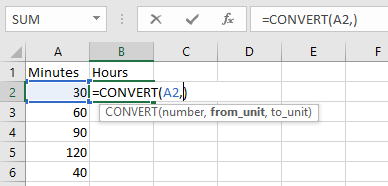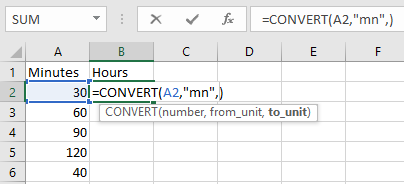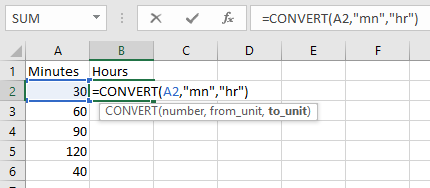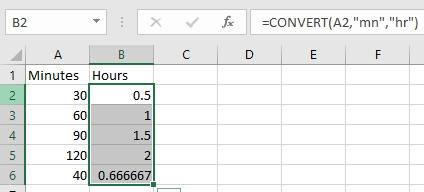# Convert Number of Minutes to Hours and Minutes in Microsoft Excel

Excel stores Date and Time in number form. To convert hours from one standard time format to others, we can use the CONVERT Function.

CONVERT function converts a number from one measurement system to another.
Syntax:

 =CONVERT(number,from_unit,to_unit)

Let’s do this with an example

In column A, we have Minutes &we need to convert the number of Minutes to Hours in column B.The second argument in the Convert Function is from_unit which is “mn” for Minutes.In the third argument enter or select to_unit as “hr” for Hour.Applying the above formula

 =CONVERT(A2,"mn","hr")Copy the formula in other cells, select the cells taking the first cell where the formula is already applied, use shortcut key CTRL + D.

Desired output will be like shown belowHope you learned how to use conversion using CONVERT function. You can perform these functions Excel 2013 and 2010. Explore more articles on conversion here. Please let us know if you have any unresolved query. We will help you.

Related Articles:

Stone to lbs

minutes to hours and minutes

Convert Date and Time from GMT to CST

Popular Articles:

50 Excel Shortcut to Increase Your Productivity

The VLOOKUP Function in Excel

COUNTIF in Excel 2016

How to Use SUMIF Function in Excel

## Users are saying about us...

1.thanks

2.Great job on explaining. Solved my problem.

What I cant figure out is how do I add a column with "hours" and a column with "minutes" to sum both into "hours & minutes"?

•175 clock rings (i.e. Begin tour - 0700: out for lunch- 1130: inform lunch - 1230: end of day 2:30
How do I convert these times to hours worked?

3.Thank you, was struggling how to convert from decimal to Hrs & minutes and your solution is both simple & elegant

4.Thanks for the great guide

5.www.scottishlgbt.org

It works really well for me

6.thanks very uselful information but if number come like 17.75(in hrs) and we know that 60 mins = 1hr, how can same be rounded off or to put condition that if after ". decimal" number is greater than 60 it will add in hr. like 17.75 = 18.15, is that can be done.

7.Excellent, clear and concise explanations. Thank you for for the help.

8.Álvaro González

I most likely misunderstood the article altogether but the only way I found to convert minutes to hours and minutes was:

1. Convert to days:

=A1/(24*60)

1. Apply this custom format:

[h]:mm;@

•Thank you! It was very helpful!

9.Thank you! It was very helpful!

10.assuming your number of minutes is in cell L16 then

= (INT(L16/60)) & " hours " & ROUND((((L16/60)-INT(L16/60))*60),0) & " minutes"

11.Need to convert 10305 minutes in Hours....... if i use this formula......then it comes 171.75
Now its look 171 hrs and 75 minutes. but 75 minutes means 01 hrs and 15 min. so please suggest

•nah.. its 171 hours and 75% of an hour (or 45 minutes)
=INT(10305/60)&" Hours & "&((10305/60)-INT(10305/60))*60&" Minutes"
171 Hours & 45 Minutes

Substitute the static 10305 value with a cell location to fit your needs...

12.WHY IS NO ONE JUST DIVIDING BY 60

•You can do that too Marcus. But Excel provides a dedicated function for conversion of units to other units, and the motive is to teach about that function.

13.WHERE do I access the "convert" tab location, needed to begin this process?

Terms and Conditions of use

The applications/code on this site are distributed as is and without warranties or liability. In no event shall the owner of the copyrights, or the authors of the applications/code be liable for any loss of profit, any problems or any damage resulting from the use or evaluation of the applications/code.